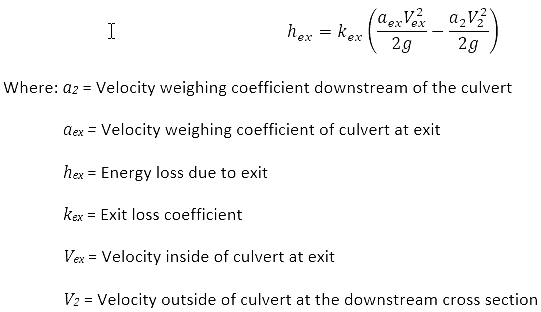1. Home
2. Knowledge Base
3. GeoHECRAS
4. HEC‑RAS Culvert FHWA Full Flow Computations
1. Home
2. Knowledge Base
3. HEC-RAS Computations
4. HEC‑RAS Culvert FHWA Full Flow Computations

# HEC‑RAS Culvert FHWA Full Flow ComputationsCulverts are made up of an inlet where water flows into the culvert, a barrel, which is the closed conduit portion of the culvert, and an outlet where water flows out of the culvert. Culvert designs that convey water under roadways with optimal capacity and minimal headwater build up are generally preferred to alternative designs. To ensure optimal flow-through, the culvert should be designed to minimize losses incurred at the inlet, barrel, and outlet. To learn more about key culvert design considerations, refer to this article in our knowledge base.

For culverts flowing full, the total head loss (or energy loss) through the culvert is measured in feet (or meters).

The head loss, HL, is computed using the following formula:## Entrance Loss

The entrance loss is computed as a coefficient multiplied by the absolute velocity head of the flow inside the culvert at the upstream end. The entrance loss for the culvert is computed as:The coefficient entered in the above equation is multiplied by the velocity head inside the culvert at the upstream end. This value represents the amount of energy loss that occurs as flow transitions from the upstream cross section to the interior of the culvert barrel. This coefficient is used in the outlet control computations and will not affect inlet control computations, as they are performed with the Federal Highway Inlet Control equations directly.

## Friction Loss

The friction loss in the culvert is computed using Manning’s formula, which is expressed as follows:## Exit Loss

The exit energy loss is computed as a coefficient multiplied by the change in velocity head from just inside the culvert, at the downstream end, to outside of the culvert at the downstream end. The equation for computing exit losses is as follows:The coefficient entered in the above equation is multiplied by the change in velocity head from inside the culvert to outside the culvert at the downstream end. This value represents the energy loss that occurs as water exits the culvert. This coefficient is used in the outlet control computations.